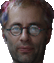<% option explicit dim Title(20) Title(1) = "Silence" Title(2) = "Self-Portrait" Title(3) = "Post Factum" Title(4) = "Obstacles" Title(5) = "I Still Remember" Title(6) = "Farclosure" Title(7) = "Eating Sky" Title(8) = "Do I?" Title(9) = "Discover" Title(10) = "Disappear" Title(11) = "Bound" Title(12) = "Blood Donation" Title(13) = "Before Arrival" Title(14) = "Light My Sky" Title(15) = "This Side of That Side" Title(16) = "Every Day Matters" Title(17) = "Invite Me Twice" Title(18) = "Destination" Title(19) = "Flying In Between" Title(20) = "Explanation" dim Rows, Columns, Cells, Number(), n, RandomPic, rs, Row, Column, ColumnWidth, Picture() Rows = 4 Columns = 5 Cells = Rows * Columns ColumnWidth = int (100 / Columns) '''''''''''''''''''''''''''''''''''''''''''''''''''''''''''''''''''''''''''''''''''''''''''''''' sub RefreshNumber dim m, NewNumber(), NewNumberSize redim NewNumber (0) for m = 1 to ubound(number) if not number(m) = 0 then NewNumberSize = ubound(newnumber) + 1 redim preserve NewNumber(NewNumberSize) NewNumber(NewNumberSize) = number (m) end if next 'Number() = Cbyte(NewNumber()) redim Number (ubound(newnumber)) for m = 1 to ubound(newnumber) Number (m) = NewNumber (m) next end sub '''''''''''''''''''''''''''''''''''''''''''''''''''''''''''''''''''''''''''''''''''''''''''''''' redim Number(Cells) for n = 1 to Cells Number (n) = n next reDim Picture(Cells) randomize for n = 1 to Cells RandomPic = int (rnd * ubound(number) + 1) Picture (n) = number(randompic) number (randompic) = 0 RefreshNumber next %> Silence by A. Molotkov

Silence
by A. Molotkov

<% for Row = 1 to Rows %> <% for Column = 1 to Columns %> <% next %> <% next %><% = Title(Picture((Row - 1) * Columns + Column)) %>

Click on an image to expand.  Refresh the page to reorder images.

Advanced viewing options (IE 4+ only) * About Silence * "Reality", a photo gallery by AM
"Not From Around Now (Poetry for a Small Choir)",  illustrated collection by AM
Contact A. Molotkov *
A. Molotkov's home page * A. Molotkov's art at Discord Aggregate Art Store